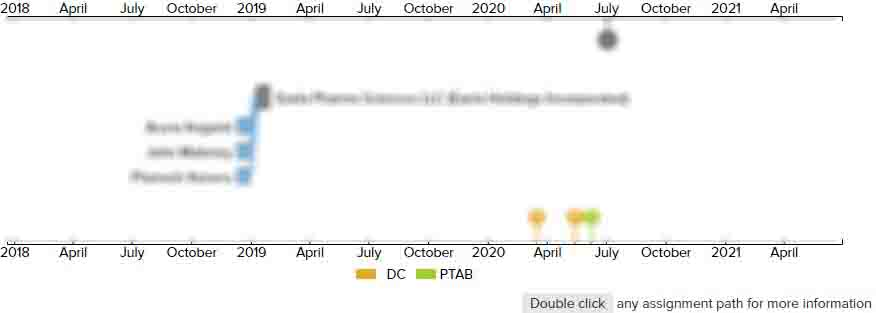# AC-to-AC converter and method for converting a first frequency AC-voltage to a second frequency AC-voltage

• US 9,099,933 B2
• Filed: 11/21/2011
• Issued: 08/04/2015
• Est. Priority Date: 12/08/2010
• Status: Active Grant
##### First Claim
Patent Images

1. A wind turbine comprising:

• an electric generator having at least a first output terminal and a second output terminal for outputting a variable frequency power signal;

a rotor for driving the electric generator;

an AC-to-AC-converter for converting a first frequency AC-voltage to a second frequency AC-voltage, the converter comprising;

a first converter section including at least a first input terminal and a second input terminal and including a first DC-output node and a second DC-output node, the first converter section being adapted for converting the first frequency AC-voltage applied between the first input terminal and the second input terminal to a DC-voltage between the first DC-output node and the second DC-output node;

a second converter section including a first DC-input node connected to the first DC-output node and including a second DC-input node connected to the second DC-output node and including at least two second output terminals, the second converter section being adapted for converting the DC-voltage between the first DC-input node and the second DC-input node to the second frequency AC-voltage between the at least two second output terminals;

a DC-link overvoltage clamp coupled between the first DC-output node and the second DC-output node, the DC-link overvoltage clamp configured to clamp the DC-voltage between the first DC-output node and the second DC-output node to be below a threshold voltage;

a capacitor system coupled between the first DC-output node and the second DC-output node; and

a heat dissipater including a heat supply surface and a heat dissipation surface for dissipating heat supplied to the heat supply surface,wherein the first input terminal of the first converter section is connected to the first output terminal of the generator and the second input terminal of the first converter section is connected to the second output terminal of the generator,wherein the DC-link overvoltage clamp is arranged adjacent to the first converter section or to the second converter section,wherein the first converter section comprises at least one first power transistor in thermal contact with the heat supply surface,wherein the second converter section comprises at least one second power transistor in thermal contact with the heat supply surface,wherein the DC-link overvoltage clamp comprises a plurality of components including a third power transistor in thermal contact with the heat supply surface,wherein the first converter section, the second converter section, the DC-link overvoltage clamp, and the capacitor system are integrated into one assembly, wherein the plurality of components that comprises the DC-link overvoltage clamp is internally disposed in the one assembly,wherein the heat dissipater is integrally formed, andwherein all power transistors comprised in the first converter section, the second converter section and in the DC-link overvoltage clamp are all thermally coupled to the same heat dissipater;

the AC-to-AC-converter further comprising a DC-link-laminate system comprising a first metal surface connected to the first DC-output node and a second metal surface connected to the second DC-output node.

• ##### 1 Assignment
Timeline View
Assignment View×
×